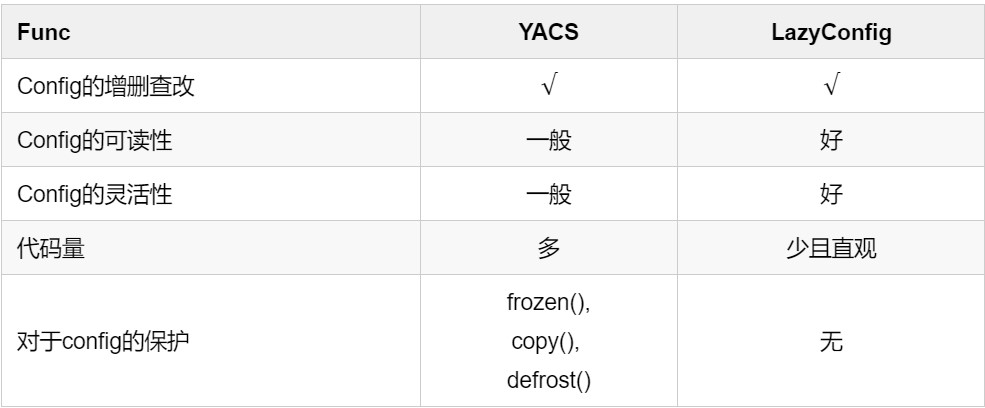## 科研视野

### 分享IDEA研究院的科研趋势观察与工作随笔

3.1、如何通过YACS创建config
3.2、Congfig System的核心需求：增删查改
3.3、如何合理地更新和保护定义好的Config
3.4、YACS小结

4.1、LazyConfig样例介绍
4.2、什么是LazyConfig中的”lazy”?
4.3、LazyConfig：在保证基本功能的前提下更加灵活

### 一、引言

detrex项目地址: https://github.com/IDEA-Research/detrex

### 二、介绍

• 方便修改大部分实验所需要的超参
• 比较方便用户管理和回溯每次实验
• 让项目结构更加清晰

### 三、YACS基本用法

3.1、如何通过YACS创建config

``MODEL:` `  BACKBONE: R50` `  NORM: BN` `NAME: Test``

``from yacs.config import CfgNode as CN` `from yacs.config import CfgNode as CN` `cfg = CN()` `cfg.NAME = "Test"` `cfg.MODEL = CN()` `cfg.MODEL.BACKBONE = "R50"` `cfg.MODEL.NORM = "BN"``

``MODEL:` `  BACKBONE: R50` `  NORM: BN` `NAME: Test``

3.2、Config System的核心需求: 增删查改

``from yacs.config import CfgNode as CN` `cfg = CN()` `cfg.NAME = "Test"` `cfg.MODEL = CN()` `cfg.MODEL.BACKBONE = "R50"` `cfg.MODEL.NORM = "BN"``

``# 1. 访问某个参数` `print(cfg.NAME)` `>>> Test` `# 2. 修改某个参数` `cfg.NAME = "Update"` `print(cfg.NAME)` `>>> Update` `# 3. 新增一个参数` `cfg.NEW_PARAM = "New"` `print(cfg.NEW_PARAM)` `>>> New``

3.3、如何合理地更新和保护定义好的Config

CfgNode.clone(): 返回一份复制的config，对其进行修改不会影响你最初定义的config内容：

``from yacs.config import CfgNode as CN` `cfg = CN()` `cfg.NAME = "Test"` `# 将cfg中的内容完整地复制给new_cfg` `new_cfg = cfg.clone()` `print(new_cfg.NAME)` `>>> Test` `# 对new_cfg进行修改, 不会影响原cfg中的参数` `new_cfg.NAME = "New"` `print(new_cfg.NAME)` `>>> New` `print(cfg.NAME)` `>>> Test``

.clone()方法，一方面可以作为对原来config的一种保护，另一方面也是快速创建一个新的config的方式

CfgNode.freeze()CfgNode.defrost(): 作为一个开关, 可以保护定义好的params不可被修改对于配置参数的保护，yacs提供了另一种方法，即可以通过freeze()方法冻结整个配置系统，使其在freeze之后无法进行任何修改，这也保证了整个过程中我们的配置不会有任何的改动。

``from yacs.config import CfgNode as CN` `cfg = CN()` `cfg.NAME = "Test"` `# 保护cfg下定义的所有参数不可被修改` `cfg.freeze()` `cfg.NAME = "New"` `>>> AttributeError: Attempted to set NAME to New, but CfgNode is immutable` `# 可以通过defrost让超参重新变得可修改` `cfg.defrost()` `cfg.NAME = "New"` `print(cfg.NAME)` `>>> New``

3.4、YACS小结

yacs作为一个极其lightweight的config system，可以说是麻雀虽小五脏俱全。其中一些基本的应用如果熟悉了，有助于帮助到大家平时的实验：

• config的增删查改
• 如何dump出config的内容到某个.yaml文件
• 如何通过命令行传参修改config中的内容，可以直接通过bash修改参数

### 四、LazyConfig基本用法

4.1、LazyConfig样例介绍

YACS Config System：

``import torch.nn as nn` `from yacs.config import CfgNode as CN` `# 创建一个config node, 并且罗列我们需要控制的参数` `cfg = CN()` `cfg.in_channels = 16` `cfg.out_channels = 16` `cfg.kernel_size = 3` `cfg.stride = 1` `cfg.padding = 1` `# 通过cfg传入超参, 实例化一个卷积层` `conv_layer = nn.Conv2d(` `    in_channels=cfg.in_channels,` `    out_channels=cfg.out_channels,` `    kernel_size=cfg.kernel_size,` `    stride=cfg.stride,` `    padding=cfg.padding,` `)` `# 打印cfg` `print(cfg)` `>>> in_channels: 16` `    kernel_size: 3` `    out_channels: 16` `    padding: 1` `    stride: 1` `# 打印conv_layer` `print(conv_layer)` `>>> Conv2d(16, 16, kernel_size=(3, 3), stride=(1, 1), padding=(1, 1))``

LazyConfig System

``import torch.nn as nn` `from detectron2.config import LazyCall, instantiate` `# 通过LazyCall创建一个config对象` `conv_config = LazyCall(nn.Conv2d)(in_channels=16, out_channels=16, kernel_size=3, stride=1, padding=1)` `# 通过instantiate实例化` `conv_layer = instantiate(conv_config)` `# 打印conv_layer` `print(conv_layer)` `>>> Conv2d(16, 16, kernel_size=(3, 3), stride=(1, 1), padding=(1, 1))``

4.2、什么是LazyConfig中的 “lazy” ？

LazyConfig中的核心思想是Lazy，Lazy表示一种延迟状态。这里的表述可能会让人云里雾里。我们结合上一小节的example，对lazyconfig中的核心思想做进一步解释，我们可以看到在上文创建卷积层的这个example中，我们新接触到了两个函数，LazyCall以及instantiate, 这也是LazyConfig中的核心用法，我们和直接通过nn.Conv2d创建一层卷积层进行一下简单的对比：

``import torch.nn as nn` `from detectron2.config import LazyCall, instantiate` `# 通过LazyCall创建一个config对象` `conv_config = LazyCall(nn.Conv2d)(in_channels=16, out_channels=16, kernel_size=3, stride=1, padding=1)` `conv_layer = instantiate(conv_config)` `# 直接通过nn.Conv2d创建conv_layer` `conv_layer = nn.Conv2d(in_channels=16, out_channels=16, kernel_size=3, stride=1, padding=1)``

1.通过LazyCall将需要实例化的对象包裹一下，传入对应的参数
2.通过instantiate来进行实例化

``import torch.nn as nn` `from detectron2.config import LazyCall, instantiate` `# 通过LazyCall创建一个config对象` `conv_config = LazyCall(nn.Conv2d)(in_channels=16, out_channels=16, kernel_size=3, stride=1, padding=1)` `print(conv_config)` `>>> {'in_channels': 16, 'out_channels': 16, 'kernel_size': 3, 'stride': 1, 'padding': 1, '_target_': <class 'torch.nn.modules.conv.Conv2d'>}``

• 一个特殊的名为_target_的key，表示我们需要实例化的类，在这个例子中是torch.nn.modules.conv.Conv2d
• 实例化_target_所需要的所有的参数，都以key-value的形式保存在这个DictConfig对象中

• 我们可以在实例化这个对象前，对其任意的value进行修改，甚至是_target_的value
• 可读性好，从读者阅读的角度而言，和直接调用nn.Conv2d的区别不大，只是额外wrap了一层，方便我们可以通过config去控制修改超参

4.3、LazyConfig：在保证基本功能的前提下更加灵活

config的修改：

``import torch.nn as nn` `from detectron2.config import LazyCall, instantiate` `# 通过LazyCall创建一个config对象` `conv_config = LazyCall(nn.Conv2d)(in_channels=16, out_channels=16, kernel_size=3, stride=1, padding=1)` `# 修改kernel_size` `conv_config.kernel_size = 5` `# 实例化这个对象, 得到kernel_size=5的卷积层` `conv_layer = instantiate(conv_config)` `print(conv_layer)` `>>> Conv2d(16, 16, kernel_size=(5, 5), stride=(1, 1), padding=(1, 1))``

config的增加

``import torch.nn as nn` `from detectron2.config import LazyCall, instantiate` `# 通过LazyCall创建一个config对象` `conv_config = LazyCall(nn.Conv2d)(in_channels=16, out_channels=16, kernel_size=3, stride=1, padding=1)` `# 新增一个groups参数, 用来控制分组卷积的组数` `conv_config.groups = 16` `# 实例化这个对象, 得到groups=16的分组卷积` `conv_layer = instantiate(conv_config)` `print(conv_layer)` `>>> Conv2d(16, 16, kernel_size=(3, 3), stride=(1, 1), padding=(1, 1), groups=16)``

LazyCall的flexible也会带来一个问题，当你新增一个不属于需要实例化的那个类的参数的时候，会报相应的错误，LazyCall不存在超参检查的机制，需要用户对传入的参数足够了解：

``import torch.nn as nn` `from detectron2.config import LazyCall, instantiate` `# 通过LazyCall创建一个config对象` `conv_config = LazyCall(nn.Conv2d)(in_channels=16, out_channels=16, kernel_size=3, stride=1, padding=1)` `# 新增了一个不属于nn.Conv2d的参数并且实例化` `conv_config.embed_dim = 16` `conv_layer = instantiate(conv_config)``

``Error when instantiating torch.nn.modules.conv.Conv2d!` `TypeError: __init__() got an unexpected keyword argument 'embed_dim'``

config的删除：

``import torch.nn as nn` `from detectron2.config import LazyCall, instantiate` `# 通过LazyCall创建一个config对象` `conv_config = LazyCall(nn.Conv2d)(in_channels=16, out_channels=16, kernel_size=3, stride=1, padding=1)` `# 删除padding这个参数` `del conv_config.padding` `# 实例化这个对象` `conv_layer = instantiate(conv_config)` `print(conv_layer)` `>>> Conv2d(16, 16, kernel_size=(3, 3), stride=(1, 1))``

``import torch.nn as nn` `from detectron2.config import LazyCall, instantiate` `# 通过LazyCall创建一个config对象` `conv_config = LazyCall(nn.Conv2d)(in_channels=16, out_channels=16, kernel_size=3, stride=1, padding=1)` `# 修改target参数, 将实例化的对象改为Conv1D` `conv_config._target_ = nn.Conv1D` `# 实例化这个对象, 得到groups=16的分组卷积` `conv_layer = instantiate(conv_config)` `print(conv_layer)` `>>> Conv1d(16, 16, kernel_size=(3,), stride=(1,), padding=(1,))``

### 五、LazyConfig与YACS的简单对比

``import torch.nn as nn` `from yacs.config import CfgNode as CN` `# 创建一个config node, 并且罗列我们需要控制的参数` `cfg = CN()` `# 创建一个子config node来控制Conv2d所需要的参数` `cfg.CONV = CN()` `cfg.CONV.in_channels = 16` `cfg.CONV.out_channels = 16` `cfg.CONV.kernel_size = 3` `cfg.CONV.stride = 1` `cfg.CONV.padding = 1` `# 创建一个子config node来控制MultiheadAttention参数` `cfg.ATTN = CN()` `cfg.ATTN.num_heads = 8` `cfg.ATTN.embed_dim = 256` `# 创建一个参数来控制我们需要创建的模型` `cfg.MODEL = "conv"` `# 构建一个build_model()函数来根据config返回我们需要的模型` `def build_model(cfg):` `if cfg.MODEL == "conv":` `model = nn.Conv2d(` `in_channels=cfg.CONV.in_channels,` `out_channels=cfg.CONV.out_channels,` `kernel_size=cfg.CONV.kernel_size,` `stride=cfg.CONV.stride,` `padding=cfg.CONV.padding,` `)` `elif cfg.MODEL == "attn":` `model = nn.MultiheadAttention(` `embed_dim=cfg.ATTN.embed_dim,` `num_heads=cfg.ATTN.num_heads` `)` `else:` `raise NotImplementedError("only implement conv and attn")` `return model` `model = build_model(cfg)` `print(model)` `>>> Conv2d(16, 16, kernel_size=(3, 3), stride=(1, 1), padding=(1, 1))``

``import torch.nn as nn` `from detectron2.config import LazyCall, instantiate` `# 通过LazyCall创建一个conv config对象` `conv_config = LazyCall(nn.Conv2d)(in_channels=16, out_channels=16, kernel_size=3, stride=1, padding=1)` `# 通过LazyCall构建一个attn config对象` `attn_config = LazyCall(nn.MultiheadAttention)(embed_dim=256, num_heads=8)` `# 根据我们的需要实例化对应的模型` `model = instantiate(attn_config)``

### 六、总结

YACS与LazyConfig在功能与灵活性上的比较：• 配置参数的增删查改
• 如何合并多组配置参数
• 如何合并不同格式的config配置, merge from List, Dict, .yaml ,e.g.
• 如何通过命令行修改参数

# YACS vs LazyConfig: Detectron2下的两代配置系统的介绍

• 分享：
detectron2 作为视觉领域的codebase标杆，在配置系统上做了很精细的设计，本文作为一篇引子，能够帮助大家更好地上手detectron2以及detrex

3.1、如何通过YACS创建config
3.2、Congfig System的核心需求：增删查改
3.3、如何合理地更新和保护定义好的Config
3.4、YACS小结

4.1、LazyConfig样例介绍
4.2、什么是LazyConfig中的”lazy”?
4.3、LazyConfig：在保证基本功能的前提下更加灵活

### 一、引言

detrex项目地址: https://github.com/IDEA-Research/detrex

### 二、介绍

• 方便修改大部分实验所需要的超参
• 比较方便用户管理和回溯每次实验
• 让项目结构更加清晰

### 三、YACS基本用法

3.1、如何通过YACS创建config

``MODEL:` `  BACKBONE: R50` `  NORM: BN` `NAME: Test``

``from yacs.config import CfgNode as CN` `from yacs.config import CfgNode as CN` `cfg = CN()` `cfg.NAME = "Test"` `cfg.MODEL = CN()` `cfg.MODEL.BACKBONE = "R50"` `cfg.MODEL.NORM = "BN"``

``MODEL:` `  BACKBONE: R50` `  NORM: BN` `NAME: Test``

3.2、Config System的核心需求: 增删查改

``from yacs.config import CfgNode as CN` `cfg = CN()` `cfg.NAME = "Test"` `cfg.MODEL = CN()` `cfg.MODEL.BACKBONE = "R50"` `cfg.MODEL.NORM = "BN"``

``# 1. 访问某个参数` `print(cfg.NAME)` `>>> Test` `# 2. 修改某个参数` `cfg.NAME = "Update"` `print(cfg.NAME)` `>>> Update` `# 3. 新增一个参数` `cfg.NEW_PARAM = "New"` `print(cfg.NEW_PARAM)` `>>> New``

3.3、如何合理地更新和保护定义好的Config

CfgNode.clone(): 返回一份复制的config，对其进行修改不会影响你最初定义的config内容：

``from yacs.config import CfgNode as CN` `cfg = CN()` `cfg.NAME = "Test"` `# 将cfg中的内容完整地复制给new_cfg` `new_cfg = cfg.clone()` `print(new_cfg.NAME)` `>>> Test` `# 对new_cfg进行修改, 不会影响原cfg中的参数` `new_cfg.NAME = "New"` `print(new_cfg.NAME)` `>>> New` `print(cfg.NAME)` `>>> Test``

.clone()方法，一方面可以作为对原来config的一种保护，另一方面也是快速创建一个新的config的方式

CfgNode.freeze()CfgNode.defrost(): 作为一个开关, 可以保护定义好的params不可被修改对于配置参数的保护，yacs提供了另一种方法，即可以通过freeze()方法冻结整个配置系统，使其在freeze之后无法进行任何修改，这也保证了整个过程中我们的配置不会有任何的改动。

``from yacs.config import CfgNode as CN` `cfg = CN()` `cfg.NAME = "Test"` `# 保护cfg下定义的所有参数不可被修改` `cfg.freeze()` `cfg.NAME = "New"` `>>> AttributeError: Attempted to set NAME to New, but CfgNode is immutable` `# 可以通过defrost让超参重新变得可修改` `cfg.defrost()` `cfg.NAME = "New"` `print(cfg.NAME)` `>>> New``

3.4、YACS小结

yacs作为一个极其lightweight的config system，可以说是麻雀虽小五脏俱全。其中一些基本的应用如果熟悉了，有助于帮助到大家平时的实验：

• config的增删查改
• 如何dump出config的内容到某个.yaml文件
• 如何通过命令行传参修改config中的内容，可以直接通过bash修改参数

### 四、LazyConfig基本用法

4.1、LazyConfig样例介绍

YACS Config System：

``import torch.nn as nn` `from yacs.config import CfgNode as CN` `# 创建一个config node, 并且罗列我们需要控制的参数` `cfg = CN()` `cfg.in_channels = 16` `cfg.out_channels = 16` `cfg.kernel_size = 3` `cfg.stride = 1` `cfg.padding = 1` `# 通过cfg传入超参, 实例化一个卷积层` `conv_layer = nn.Conv2d(` `    in_channels=cfg.in_channels,` `    out_channels=cfg.out_channels,` `    kernel_size=cfg.kernel_size,` `    stride=cfg.stride,` `    padding=cfg.padding,` `)` `# 打印cfg` `print(cfg)` `>>> in_channels: 16` `    kernel_size: 3` `    out_channels: 16` `    padding: 1` `    stride: 1` `# 打印conv_layer` `print(conv_layer)` `>>> Conv2d(16, 16, kernel_size=(3, 3), stride=(1, 1), padding=(1, 1))``

LazyConfig System

``import torch.nn as nn` `from detectron2.config import LazyCall, instantiate` `# 通过LazyCall创建一个config对象` `conv_config = LazyCall(nn.Conv2d)(in_channels=16, out_channels=16, kernel_size=3, stride=1, padding=1)` `# 通过instantiate实例化` `conv_layer = instantiate(conv_config)` `# 打印conv_layer` `print(conv_layer)` `>>> Conv2d(16, 16, kernel_size=(3, 3), stride=(1, 1), padding=(1, 1))``

4.2、什么是LazyConfig中的 “lazy” ？

LazyConfig中的核心思想是Lazy，Lazy表示一种延迟状态。这里的表述可能会让人云里雾里。我们结合上一小节的example，对lazyconfig中的核心思想做进一步解释，我们可以看到在上文创建卷积层的这个example中，我们新接触到了两个函数，LazyCall以及instantiate, 这也是LazyConfig中的核心用法，我们和直接通过nn.Conv2d创建一层卷积层进行一下简单的对比：

``import torch.nn as nn` `from detectron2.config import LazyCall, instantiate` `# 通过LazyCall创建一个config对象` `conv_config = LazyCall(nn.Conv2d)(in_channels=16, out_channels=16, kernel_size=3, stride=1, padding=1)` `conv_layer = instantiate(conv_config)` `# 直接通过nn.Conv2d创建conv_layer` `conv_layer = nn.Conv2d(in_channels=16, out_channels=16, kernel_size=3, stride=1, padding=1)``

1.通过LazyCall将需要实例化的对象包裹一下，传入对应的参数
2.通过instantiate来进行实例化

``import torch.nn as nn` `from detectron2.config import LazyCall, instantiate` `# 通过LazyCall创建一个config对象` `conv_config = LazyCall(nn.Conv2d)(in_channels=16, out_channels=16, kernel_size=3, stride=1, padding=1)` `print(conv_config)` `>>> {'in_channels': 16, 'out_channels': 16, 'kernel_size': 3, 'stride': 1, 'padding': 1, '_target_': <class 'torch.nn.modules.conv.Conv2d'>}``

• 一个特殊的名为_target_的key，表示我们需要实例化的类，在这个例子中是torch.nn.modules.conv.Conv2d
• 实例化_target_所需要的所有的参数，都以key-value的形式保存在这个DictConfig对象中

• 我们可以在实例化这个对象前，对其任意的value进行修改，甚至是_target_的value
• 可读性好，从读者阅读的角度而言，和直接调用nn.Conv2d的区别不大，只是额外wrap了一层，方便我们可以通过config去控制修改超参

4.3、LazyConfig：在保证基本功能的前提下更加灵活

config的修改：

``import torch.nn as nn` `from detectron2.config import LazyCall, instantiate` `# 通过LazyCall创建一个config对象` `conv_config = LazyCall(nn.Conv2d)(in_channels=16, out_channels=16, kernel_size=3, stride=1, padding=1)` `# 修改kernel_size` `conv_config.kernel_size = 5` `# 实例化这个对象, 得到kernel_size=5的卷积层` `conv_layer = instantiate(conv_config)` `print(conv_layer)` `>>> Conv2d(16, 16, kernel_size=(5, 5), stride=(1, 1), padding=(1, 1))``

config的增加

``import torch.nn as nn` `from detectron2.config import LazyCall, instantiate` `# 通过LazyCall创建一个config对象` `conv_config = LazyCall(nn.Conv2d)(in_channels=16, out_channels=16, kernel_size=3, stride=1, padding=1)` `# 新增一个groups参数, 用来控制分组卷积的组数` `conv_config.groups = 16` `# 实例化这个对象, 得到groups=16的分组卷积` `conv_layer = instantiate(conv_config)` `print(conv_layer)` `>>> Conv2d(16, 16, kernel_size=(3, 3), stride=(1, 1), padding=(1, 1), groups=16)``

LazyCall的flexible也会带来一个问题，当你新增一个不属于需要实例化的那个类的参数的时候，会报相应的错误，LazyCall不存在超参检查的机制，需要用户对传入的参数足够了解：

``import torch.nn as nn` `from detectron2.config import LazyCall, instantiate` `# 通过LazyCall创建一个config对象` `conv_config = LazyCall(nn.Conv2d)(in_channels=16, out_channels=16, kernel_size=3, stride=1, padding=1)` `# 新增了一个不属于nn.Conv2d的参数并且实例化` `conv_config.embed_dim = 16` `conv_layer = instantiate(conv_config)``

``Error when instantiating torch.nn.modules.conv.Conv2d!` `TypeError: __init__() got an unexpected keyword argument 'embed_dim'``

config的删除：

``import torch.nn as nn` `from detectron2.config import LazyCall, instantiate` `# 通过LazyCall创建一个config对象` `conv_config = LazyCall(nn.Conv2d)(in_channels=16, out_channels=16, kernel_size=3, stride=1, padding=1)` `# 删除padding这个参数` `del conv_config.padding` `# 实例化这个对象` `conv_layer = instantiate(conv_config)` `print(conv_layer)` `>>> Conv2d(16, 16, kernel_size=(3, 3), stride=(1, 1))``

``import torch.nn as nn` `from detectron2.config import LazyCall, instantiate` `# 通过LazyCall创建一个config对象` `conv_config = LazyCall(nn.Conv2d)(in_channels=16, out_channels=16, kernel_size=3, stride=1, padding=1)` `# 修改target参数, 将实例化的对象改为Conv1D` `conv_config._target_ = nn.Conv1D` `# 实例化这个对象, 得到groups=16的分组卷积` `conv_layer = instantiate(conv_config)` `print(conv_layer)` `>>> Conv1d(16, 16, kernel_size=(3,), stride=(1,), padding=(1,))``

### 五、LazyConfig与YACS的简单对比

``import torch.nn as nn` `from yacs.config import CfgNode as CN` `# 创建一个config node, 并且罗列我们需要控制的参数` `cfg = CN()` `# 创建一个子config node来控制Conv2d所需要的参数` `cfg.CONV = CN()` `cfg.CONV.in_channels = 16` `cfg.CONV.out_channels = 16` `cfg.CONV.kernel_size = 3` `cfg.CONV.stride = 1` `cfg.CONV.padding = 1` `# 创建一个子config node来控制MultiheadAttention参数` `cfg.ATTN = CN()` `cfg.ATTN.num_heads = 8` `cfg.ATTN.embed_dim = 256` `# 创建一个参数来控制我们需要创建的模型` `cfg.MODEL = "conv"` `# 构建一个build_model()函数来根据config返回我们需要的模型` `def build_model(cfg):` `if cfg.MODEL == "conv":` `model = nn.Conv2d(` `in_channels=cfg.CONV.in_channels,` `out_channels=cfg.CONV.out_channels,` `kernel_size=cfg.CONV.kernel_size,` `stride=cfg.CONV.stride,` `padding=cfg.CONV.padding,` `)` `elif cfg.MODEL == "attn":` `model = nn.MultiheadAttention(` `embed_dim=cfg.ATTN.embed_dim,` `num_heads=cfg.ATTN.num_heads` `)` `else:` `raise NotImplementedError("only implement conv and attn")` `return model` `model = build_model(cfg)` `print(model)` `>>> Conv2d(16, 16, kernel_size=(3, 3), stride=(1, 1), padding=(1, 1))``

``import torch.nn as nn` `from detectron2.config import LazyCall, instantiate` `# 通过LazyCall创建一个conv config对象` `conv_config = LazyCall(nn.Conv2d)(in_channels=16, out_channels=16, kernel_size=3, stride=1, padding=1)` `# 通过LazyCall构建一个attn config对象` `attn_config = LazyCall(nn.MultiheadAttention)(embed_dim=256, num_heads=8)` `# 根据我们的需要实例化对应的模型` `model = instantiate(attn_config)``

### 六、总结

YACS与LazyConfig在功能与灵活性上的比较：• 配置参数的增删查改
• 如何合并多组配置参数
• 如何合并不同格式的config配置, merge from List, Dict, .yaml ,e.g.